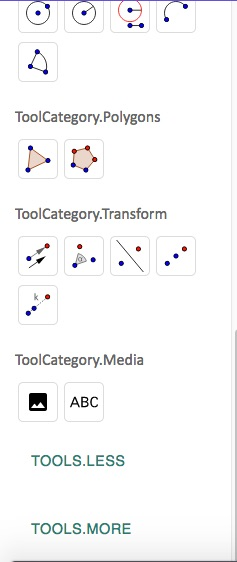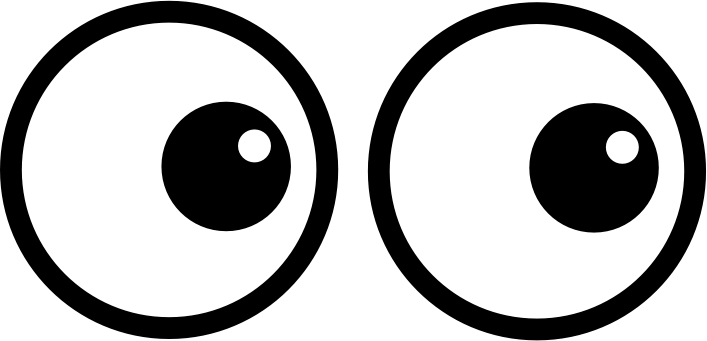# GEOM 3 | Lesson 1 | Explore (Transformations)If a triangle is drawn and rotated about the origin, does it change the size or shape of the triangle?  What if I flip it upside down? Or turn it over?  How about if it is moved to the right or the left?

What are other names for these transformations? Will any of these transformations change the shape or size?

Draw a triangle on a piece of paper and cut it out to try the transformations.  Then use graph paper, draw the $$x$$ and $$y$$ axes.  Note the coordinates of the preimage (original triangle) and the image for each of the transformations.

Another approach:  go to Geogebra  https://www.geogebra.org/geometry/wsetthbv

If you use Geogebra then transform tools in geogebra to try the transformations.  See tool box, click on the right arrow right arrow at the bottom left side to bring up the tools transformations.1).  If you may an error click on the curved arrow to undo.

2) Select a reflection (2nd from right) from the transform tool, click on the $$x$$-axis and then the triangle, the image is the reflection over the $$x$$-axis.  Then do the same over the  $$y$$-axis.  Note the coordinates of both.  You might also want to reflect it over another line say $$y = 2x$$.

3). Select a rotation, select a point in the plane, $$(0,0)$$ and then click on the triangle.  You will see pop-up that will ask you to input the angle of rotation.  Try $$90, 180$$ and $$270$$.

Are the original triangle (preimage) and its image the same size and shape?    Think about what parts of the triangle control the size and what parts control the shape.   Name all the parts that are the same size.

Can you conjecture about what happens to the coordinates for each of the transformations?